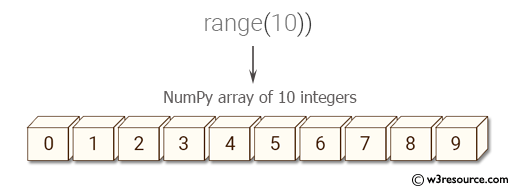﻿ NumPy: Create a NumPy array of 10 integers from a generator - w3resource# NumPy: Create a NumPy array of 10 integers from a generator

## NumPy: Array Object Exercise-85 with Solution

Write a NumPy program to create a NumPy array of 10 integers from a generator.

Pictorial Presentation:Sample Solution:-

Python Code:

``````import numpy as np
iterable = (x for x in range(10))
print(np.fromiter(iterable, np.int))
```
```

Sample Output:

```[0 1 2 3 4 5 6 7 8 9]
```

Python Code Editor:

Have another way to solve this solution? Contribute your code (and comments) through Disqus.

What is the difficulty level of this exercise?

Test your Python skills with w3resource's quiz

﻿

## Python: Tips of the Day

Set comprehension:

```>>> m = {x ** 2 for x in range(5)}
>>> m
{0, 1, 4, 9, 16}
```